Case Based Questions (MCQ)

Chapter 1 Class 12 Relation and Functions
Serial order wise

## A general election of Lok Sabha is a gigantic exercise. About 911 million people were eligible to vote and voter turnout was about 67%, the highest ever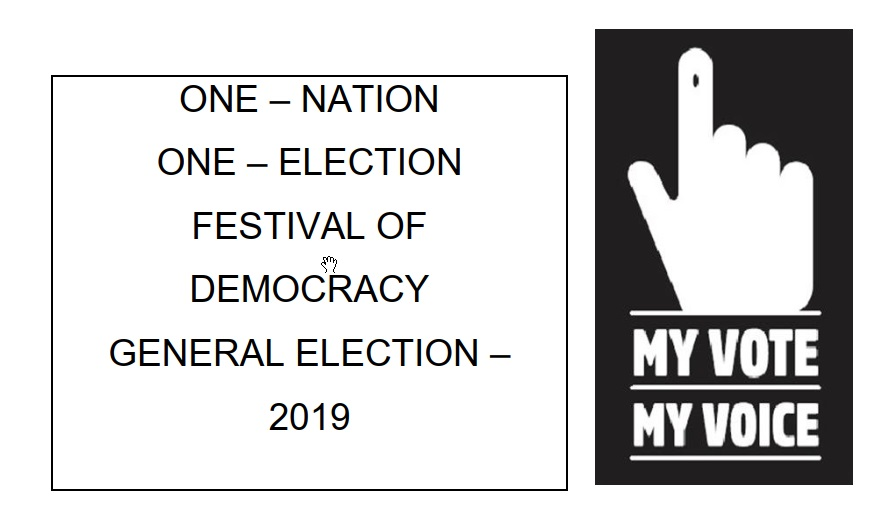googletag.cmd.push(function() { googletag.display('div-gpt-ad-1669298377854-0'); }); Let I be the set of all citizens of India who were eligible to exercise their voting right in general election held in 2019. A relation ‘R’ is defined on I as follows: R = {(𝑉 1 , 𝑉 2 ) ∶ 𝑉 1 , 𝑉 2 ∈ 𝐼 and both use their voting right in general election – 2019}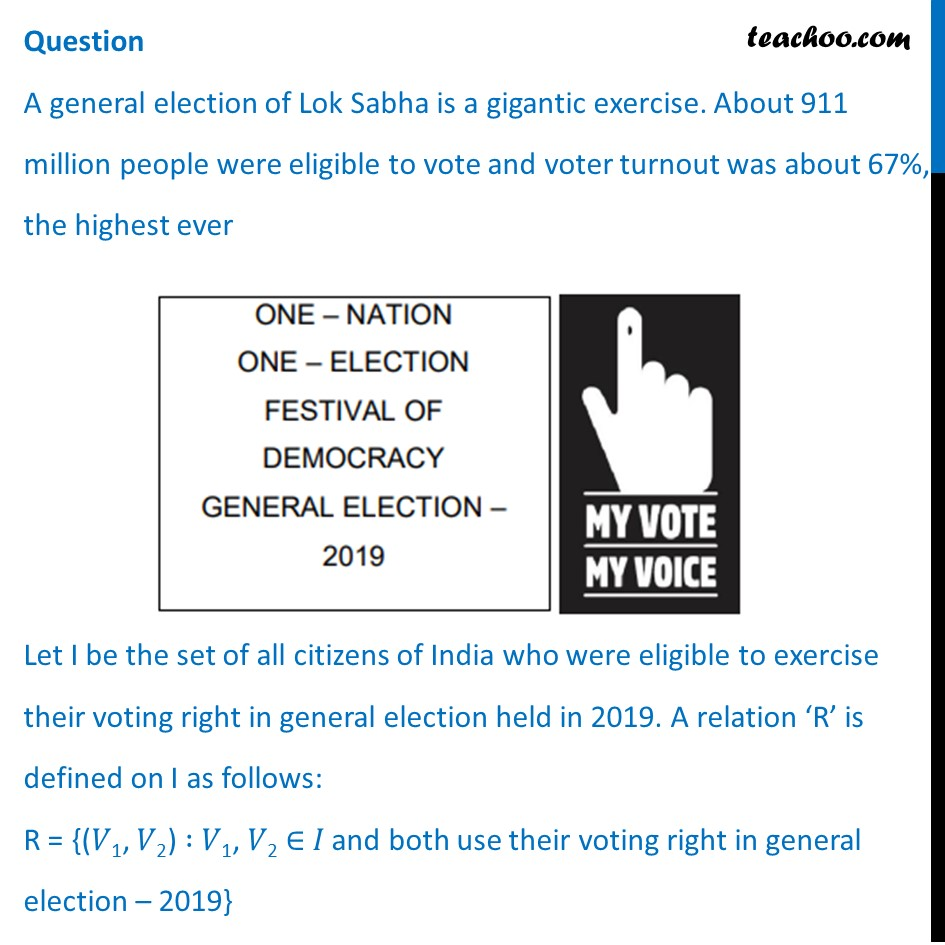## Two neighbors X & Y ∈ I. X exercised his voting right while Y did not cast her vote in general election- 2019. Which of the following is true? (a) (X, Y) ∈ R (b) (Y, X) ∈ R (c) (X, X) ∉ R (d) (X, Y) ∉ R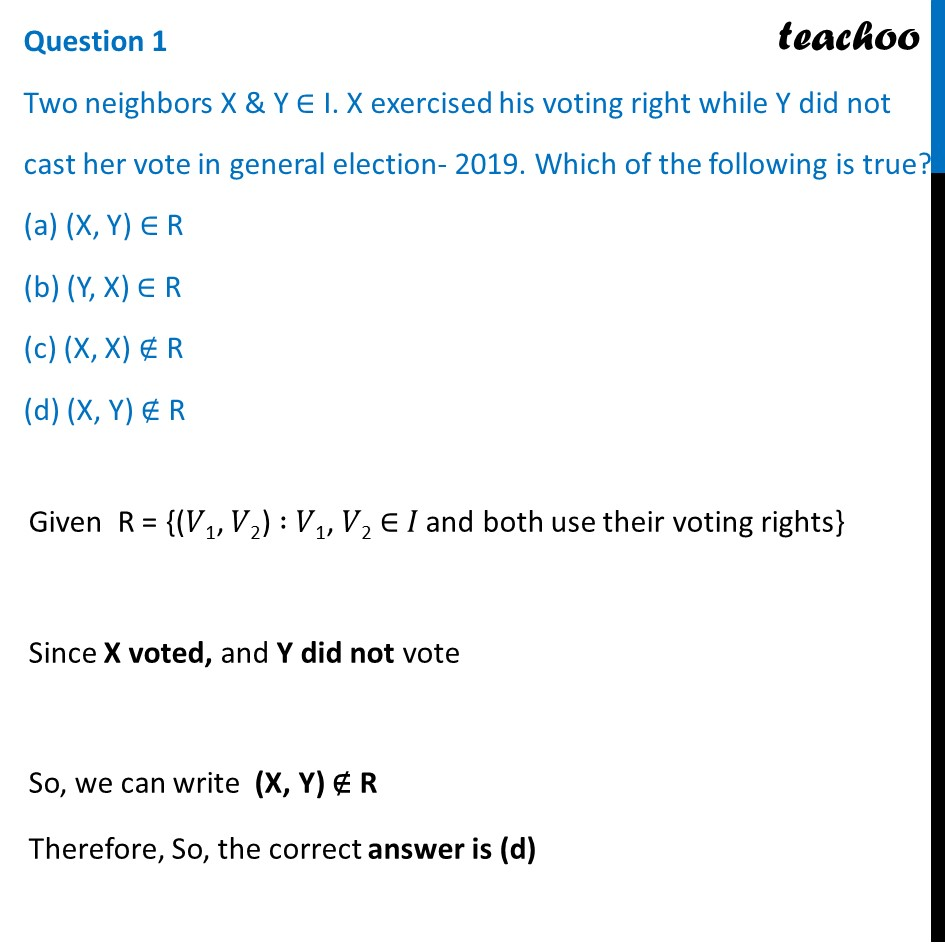## Mr. ’𝑋’ and his wife ‘𝑊’ both exercised their voting right in general election -2019. Which of the following is true? (a) both (X, W) and (W, X) ∈ R (b) (X, W) ∈ R but (W, X) ∉ R (c) both (X, W) and (W, X) ∉ R (d) (W, X) ∈ R but (X, W) ∉ R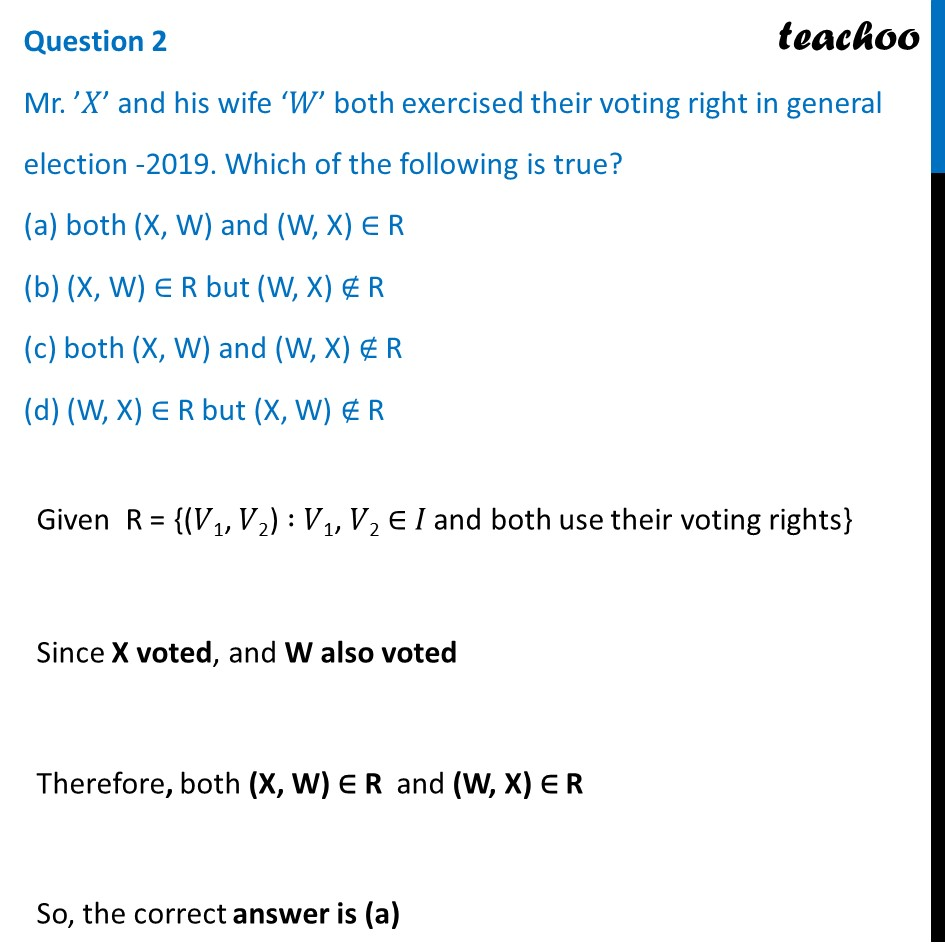## Three friends F 1 , F 2 and F 3 exercised their voting right in general election-2019, then which of the following is true? (a) (F 1 , F 2 ) ∈ R, (F 2 , F 3 ) ∈ R and (F 1 , F 3 ) ∈ R (b) (F 1 , F 2 ) ∈ R, (F 2 , F 3 ) ∈ R and (F 1 , F 3 ) ∉ R (c) (F 1 , F 2 ) ∈ R, (F 2 , F 2 ) ∈ R but (F 3 , F 3 ) ∉ R (d) (F 1 , F 2 ) ∉ R, (F 2 , F 3 ) ∉ R and (F 1 , F 3 ) ∉ R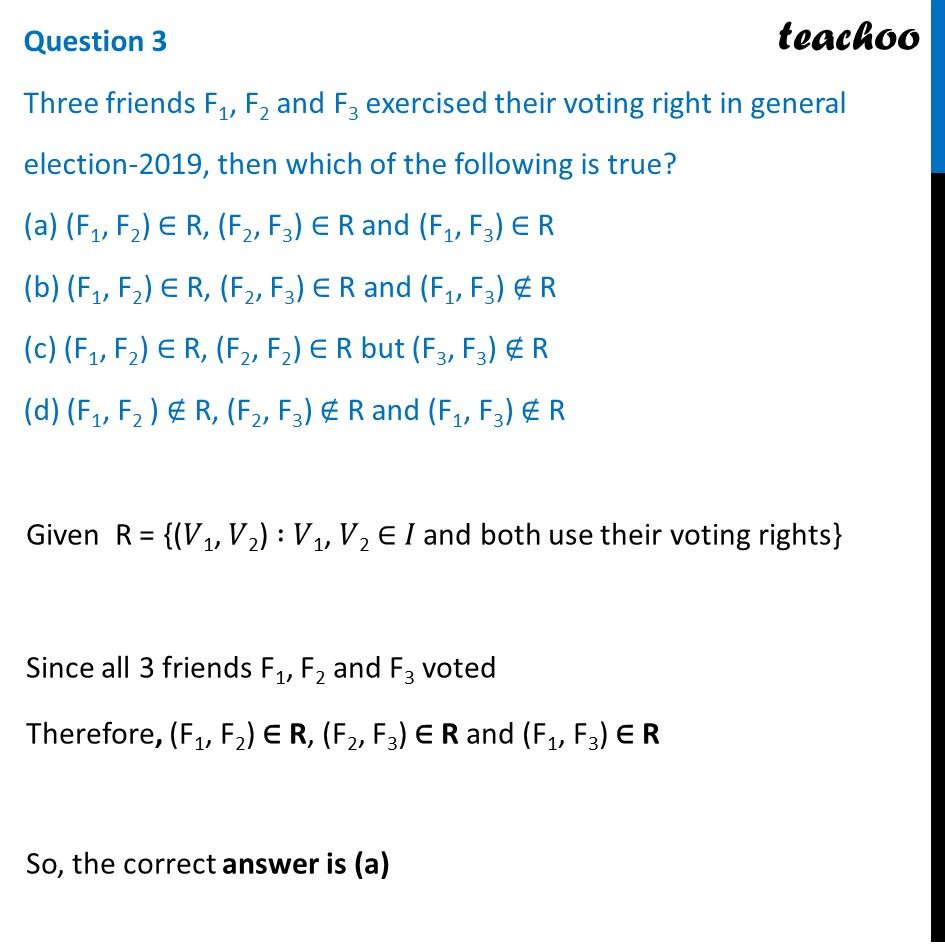## The above defined relation R is __________ (a) Symmetric and transitive but not reflexive (b) Universal relation (c) Equivalence relation (d) Reflexive but not symmetric and transitive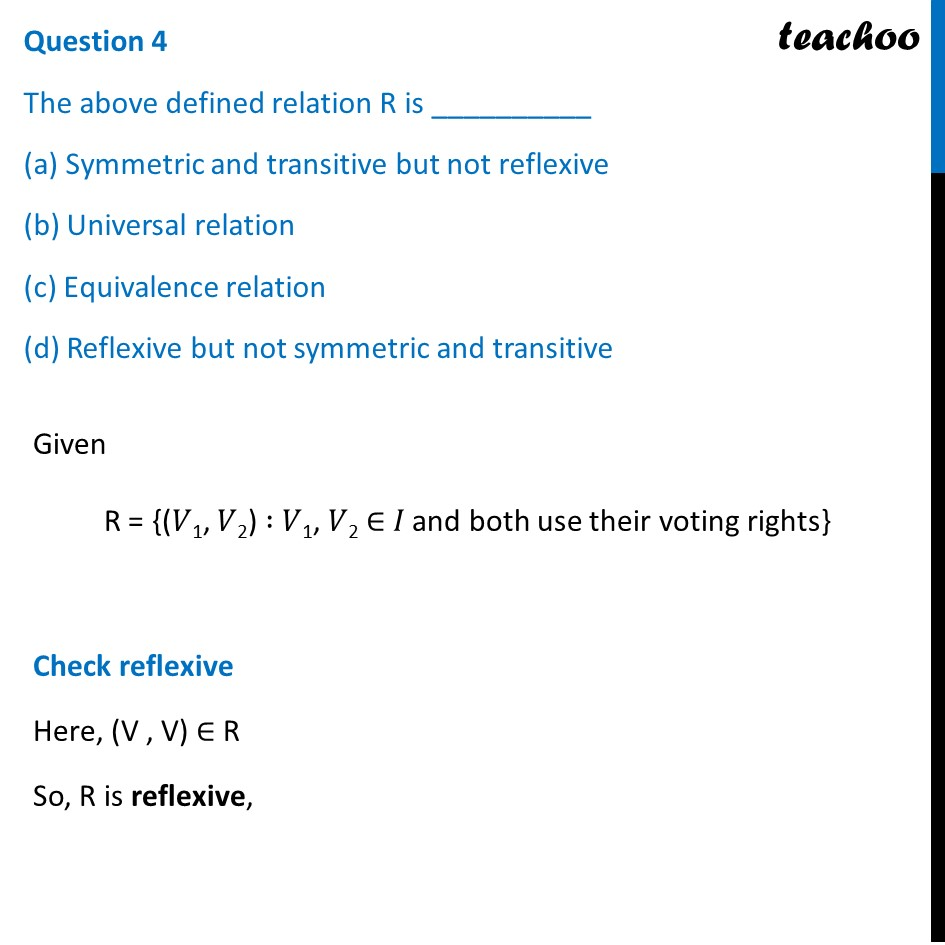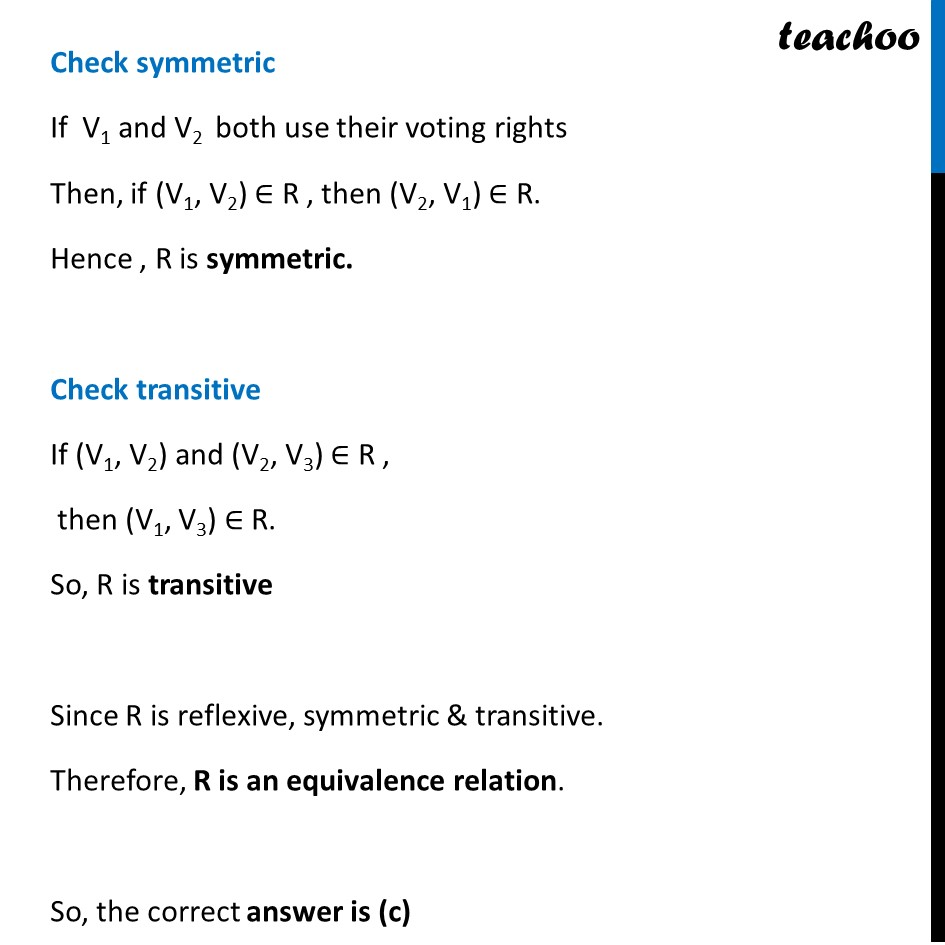## Mr. Shyam exercised his voting right in General Election – 2019, then Mr. Shyam is related to which of the following? (a) All those eligible voters who cast their votes (b) Family members of Mr. Shyam (c) All citizens of India (d) Eligible voters of India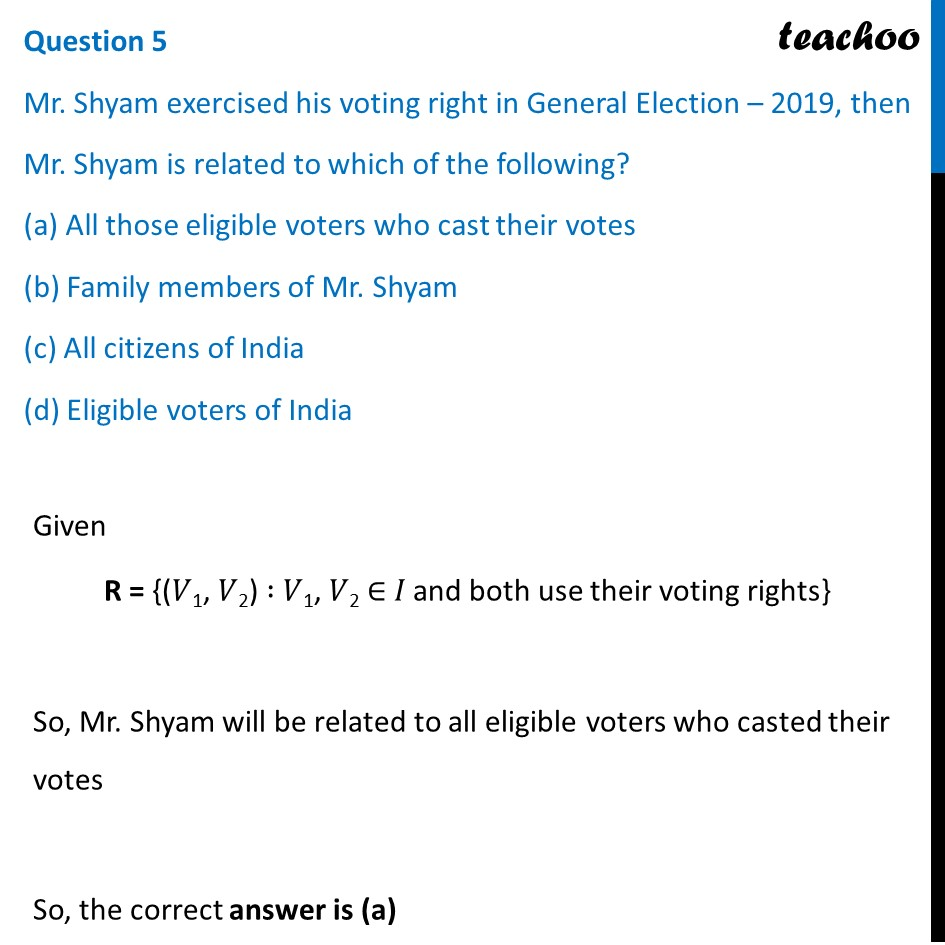Learn in your speed, with individual attention - Teachoo Maths 1-on-1 Class

### Transcript

Question A general election of Lok Sabha is a gigantic exercise. About 911 million people were eligible to vote and voter turnout was about 67%, the highest ever Let I be the set of all citizens of India who were eligible to exercise their voting right in general election held in 2019. A relation ‘R’ is defined on I as follows: R = {(𝑉1, 𝑉2) ∶ 𝑉1, 𝑉2 ∈ 𝐼 and both use their voting right in general election – 2019} Question 1 Two neighbors X & Y ∈ I. X exercised his voting right while Y did not cast her vote in general election- 2019. Which of the following is true? (a) (X, Y) ∈ R (b) (Y, X) ∈ R (c) (X, X) ∉ R (d) (X, Y) ∉ R Given R = {(𝑉1, 𝑉2) ∶ 𝑉1, 𝑉2 ∈ 𝐼 and both use their voting rights} Since X voted, and Y did not vote So, we can write (X, Y) ∉ R Therefore, So, the correct answer is (d) Question 2 Mr. ’𝑋’ and his wife ‘𝑊’ both exercised their voting right in general election -2019. Which of the following is true? (a) both (X, W) and (W, X) ∈ R (b) (X, W) ∈ R but (W, X) ∉ R (c) both (X, W) and (W, X) ∉ R (d) (W, X) ∈ R but (X, W) ∉ R Given R = {(𝑉1, 𝑉2) ∶ 𝑉1, 𝑉2 ∈ 𝐼 and both use their voting rights} Since X voted, and W also voted Therefore, both (X, W) ∈ R and (W, X) ∈ R So, the correct answer is (a) Question 3 Three friends F1, F2 and F3 exercised their voting right in general election-2019, then which of the following is true? (a) (F1, F2) ∈ R, (F2, F3) ∈ R and (F1, F3) ∈ R (b) (F1, F2) ∈ R, (F2, F3) ∈ R and (F1, F3) ∉ R (c) (F1, F2) ∈ R, (F2, F2) ∈ R but (F3, F3) ∉ R (d) (F1, F2 ) ∉ R, (F2, F3) ∉ R and (F1, F3) ∉ R Given R = {(𝑉1, 𝑉2) ∶ 𝑉1, 𝑉2 ∈ 𝐼 and both use their voting rights} Since all 3 friends F1, F2 and F3 voted Therefore, (F1, F2) ∈ R, (F2, F3) ∈ R and (F1, F3) ∈ R So, the correct answer is (a) Question 4 The above defined relation R is __________ (a) Symmetric and transitive but not reflexive (b) Universal relation (c) Equivalence relation (d) Reflexive but not symmetric and transitive Given R = {(𝑉1, 𝑉2) ∶ 𝑉1, 𝑉2 ∈ 𝐼 and both use their voting rights} Check reflexive Here, (V , V) ∈ R So, R is reflexive, Check symmetric If V1 and V2 both use their voting rights Then, if (V1, V2) ∈ R , then (V2, V1) ∈ R. Hence , R is symmetric. Check transitive If (V1, V2) and (V2, V3) ∈ R , then (V1, V3) ∈ R. So, R is transitive Since R is reflexive, symmetric & transitive. Therefore, R is an equivalence relation. So, the correct answer is (c) Question 5 Mr. Shyam exercised his voting right in General Election – 2019, then Mr. Shyam is related to which of the following? (a) All those eligible voters who cast their votes (b) Family members of Mr. Shyam (c) All citizens of India (d) Eligible voters of India Given R = {(𝑉1, 𝑉2) ∶ 𝑉1, 𝑉2 ∈ 𝐼 and both use their voting rights} So, Mr. Shyam will be related to all eligible voters who casted their votes So, the correct answer is (a)